# Dimension and rank#### Everything You Need in One Place

Homework problems? Exam preparation? Trying to grasp a concept or just brushing up the basics? Our extensive help & practice library have got you covered.#### Learn and Practice With Ease

Our proven video lessons ease you through problems quickly, and you get tonnes of friendly practice on questions that trip students up on tests and finals.#### Instant and Unlimited Help

Our personalized learning platform enables you to instantly find the exact walkthrough to your specific type of question. Activate unlimited help now!##### Intros
###### Lessons
1. Dimension and Rank Overview:
2. Dimension of a subspace
• Dimension = number of vectors in the basis
• Can we find dimension of column space and null space?
3. Rank of a Matrix
• Find the basis
• Count the # of vectors
• Shortcut = count the # of pivots
4. Dimension of the Null Space
• Find the general solution
• Put in parametric vector form
• Count the # of vectors
• Shortcut = count the # of free variables
5. The Rank Theorem
• Rank $A +$ dim $N(A) = n$
• An example of using the theorem
##### Examples
###### Lessons
1. Finding the Rank of a matrix
Find the rank of $A$ if: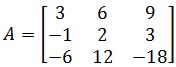1. Find a basis for the subspace spanned by the given vectors. What is the dimension of the subspace?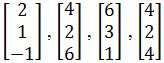1. Finding the dimension of the null space
Find the dimension of the null space of $A$ if: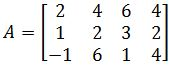1. Utilizing the Rank Theorem
You are given the matrix $A$ and the echelon form of $A$. Find the basis for the column space, and find the rank and the dimensions of the null space.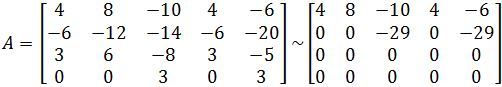1. Understanding the Theorems
Let the subspace of all solutions of $Ax=0$ have a basis consisting of four vectors, where $A$ is $4 \times 6$. What is the rank of $A$?
1. Let $A$ be a $m \times n$ matrix where the rank of $A$ is $p$. Then what is the dimension of the null space of $A$?

## Dimension and Rank

During this lesson we will learn how the different contexts in which a word such as dimension is used, can make a huge difference on the information they produce. So we will study from what is a matrix dimension and rank (from which, the first we have already learnt and used many times), what is the dimension of a subspace, and what types of these results can you obtain, depending of the subspace in question.

#### Rank and dimension linear algebra

For our first section we will concentrate on learning the concepts of rank and dimension of a matrix and of a subset. In order to understand rank, we decided to present what the term dimension means first, since the relationship between dimension and rank can be different depending on the context in which we are defining dimension in itself.

For the definition of dimension it is precise to make a few clarifications. In other lessons throughout this linear algebra course you will see how the word dimension is used differently for different scenarios. For example, when describing matrices we say that the matrix dimension is its order, in other words, the number of rows and columns contained in the matrix usually noted as $m \times n$, where $m$ is the number of rows and $n$ is the number of columns. Thus, for a matrix, its dimension is defined as $m \times n$, and the m and n quantities are its dimensions on each side.
When talking about vectors, the word dimension directly refers to the coordinate planes in which such vector can be lay down, or represented. The dimensions of a vector will be defined by the amount of different variables each corresponding to different coordinate planes contained, for example, a two-dimensional vector would be that which is defined with two different variables each corresponding to a distinct coordinate axis, such as $x$ and $y$, thus, this vector would be found in the $x-y$ plane when represented geometrically. We talked about this extensively on our lesson about the properties of subspace, since we learnt about subspaces of a span of vectors in the real coordinate space. We recommend you to go back to that lesson if you feel the concepts here may confuse you due to the usage of similar words.

On this case we will focus on the dimension of a subspace itself.
Remember, a vector subspace is a set of vectors in a particular $Rn$(which will utilize as many dimensional planes as necessary, depending on the components of the vectors in the set of the subspace) that follows the zero vector, closed under addition and closed under scalar multiplication properties.

And so, to define the dimension of a nonzero subspace $S$ we say that is the number of vectors in any basis for $S$. For example, if the basis for a subspace $S$ is defined as follows: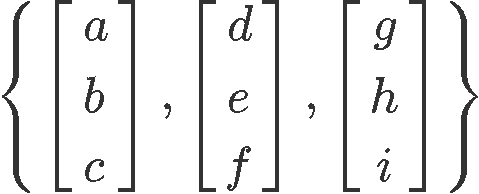We simply count the number of vectors contained in the basis and call it the dimension of subspace $S$. Therefore, for this case, we have three vectors and so, the dimension of the subspace $S$ is equal to 3. Since the dimension of a nonzero subspace $S$ is usually denoted as dim $S$, for this case we have that: dim $S = 3$
This same simple method to find the dimension of a subspace can be used with any nonzero subspace, hence, we can find the dimension of the column space and the null space of a matrix if needed.

Now let us talk about rank. The rank of a matrix is equal to the dimension of its column space. This particular concept creates an interesting (and sometimes confusing) nomenclature for dimension and rank linear algebra. Let us break this up in pieces:

The rank of a matrix is equal to the dimension of its column space (which is a subspace).
The nullity of a matrix is equal to the dimension of its null space (which is also a subspace).

So, so far these two: rank and nullity, are dimensions of subspaces (the first coming from the column space of a matrix, and the second from the null space of a matrix), but when you add them together, they produce one of the dimensions of the matrix in question: the number of columns inside the matrix, usually noted as n from the standard notation of $m \times n$.

This can be clearly explained in the set of equations below:

For any $\, m \times n \,$matrix $A$:

dim$\, C(A) = \,$rank$(A)$

dim$\, N(A) = \,$nullity$(A)$

dim$\, C(A) \, + \,$dim$N(A) = n$

same as:

rank$(A) \, + \,$nullity$(A) = n$

Where $C(A)$ = columns space of $A$, and $N(A)$ = null space of $A$.

This is what we call the rank-nullity theorem, which describes the relation between rank and dimension of null space or nullity. This theorem also allows us to see that the rank and dimension of matrix $A$ are related (notice we said dimension of matrix $A$ and NOT the dimension of a subspace, they are two different things!).

#### What is the difference between rank and dimension?

So, are dimension and rank the same?
To answer this question, we have to look to two different scenarios:

• For matrix rank and dimension of a subspace
At this point you already know that for a specific case, dimension and rank of a subspace are the same. What? How?
Well, as mentioned in our last section we know rank is a dimension of a subspace, the column space. Therefore, the difference comes from the fact that subspaces basis dimension and rank can ONLY be equal when the subspace in question is the column space of a matrix. In other words, the dimension of a subspace is the number of vectors in the basis of ANY nonzero subspace, and the rank is ONLY equal to this number, when the nonzero subspace happens to be the column space of a matrix.
And so, the difference between dimension and rank, when dimension is referring to the dimension of a subspace, is the same as the difference between dimension and nullity. They ONLY refer to the same thing when the dimension of the subspace is to the dimension of the particular subspace that produces the rank or the nullity accordingly.

• For the dimension and rank of matrix (any matrix).
In this case, we use the word dimension to refer to the dimensions of a matrix: $m \times n$.
Thus, in this case we can clearly see the difference between rank and dimension, since the rank refers to the dimension of an specific subspace from the matrix (the column space), while the word dimension here refers to the number of columns in the matrix.

In summary (and to finalize this section, which hopefully hasnt fell into redundancy) what is the difference between nullity, rank and dimension? That dimension can be taken as the quantities describing different elements, in this case, either the dimension of a subspace or the dimension of a matrix, while rank and nullity are both dimensions of a particular type of subspace from a given matrix. And so, the dimension of a subspace CAN be equal to rank or nullity, since it will be equal to rank when the subspace in question is the column space of the matrix, and it will be equal to nullity when the subspace in question is the null space of the matrix. But matrices dimension and rank are just related (NOT the same), in the same way as a matrix dimension is related to nullity, through the rank-nullity theorem.

#### Find rank and dimension

In order to find the dimension n (number of columns) of a matrix, we need to find the dimensions of the subspaces: column space and null space, or the rank and nullity of the matrix. Let us explain the steps for these two processes below, but before, let us remind you that to find n through this long method serves only as proof method of the relationship of the dimensions of these subspaces. In reality, you could just count the number of columns in a matrix and that is it. Therefore, the relevant calculations on this section is the computation of the rank and the nullity.

Finding the rank of a matrix:
1. Find the basis for the column space
1. Row reduce the matrix to echelon form (any echelon form is fine)
2. Circle the columns with pivots.
3. Go back to the original matrix and circle the columns with the same positions.
4. Use those columns to write out the basis.
2. Count the number of vectors in the basis.

Remember that our first steps computation was explained two lessons ago, when we learned about the column space of a matrix. If you have doubts on how to work it out, please go back and check the lesson on column space. You can also watch the video included on this lesson, where the rank of a matrix is introduced, for a quick review on the method.
After finding the basis for the column space of the matrix, the only thing left is to count the number of vectors in this basis to obtain the rank.

Since the process of obtaining the basis of the column space is tedious we can work the rank out through a shortcut: Counting the number of pivots in the matrix once it has been reduced to an echelon form. In that way, you don't have to write down the basis of the column space, the rank is obtained through the pivots automatically.

Finding the nullity of a matrix:
1. Find the basis for the null space.
1. Solve for $Ax = 0$.
2. Write solution in parametric vector form.
3. Write down the vectors.
2. Count the number of vectors in the basis.

Just as before, the first step of this process has been covered in a past lesson, this time, we have seen this in our lesson about null space. If you need a quick review on it, we recommend you to go back and take a look at the lesson. Also, you can follow the video included in this lesson where the process to find the dimension of null space is introduced.

Once more, we do recommend to save time and use a shortcut, in this case all we have to do is to count for the number of free variables after we have solved the equation $Ax = 0$, this will automatically provide us with the answer of the dimension of null space.

Knowing all of this, let us now learn how to find the dimension and rank of a matrix in a variety of exercise problems through the next section.

#### Rank nullity and dimension example problems:

Through the next exercises, we hope to not only help you out practicing the math involved in our concepts for today, but also aid in the clarification of any confusion that may have arisen from the repetitiveness of such concepts words.

#### Example 1

Find the rank of matrix $A$ which is defined as shown below: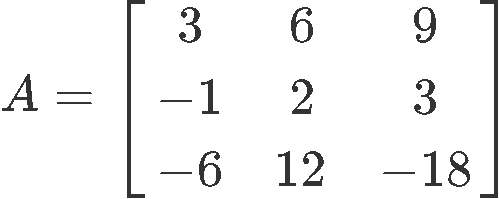For practicality, we will use the shortcut method to solve this problem, therefore, we will find the matrix echelon form and count the number of pivots available.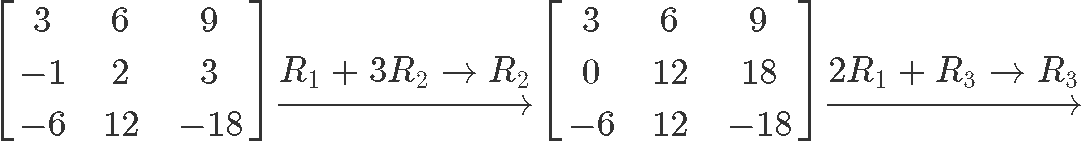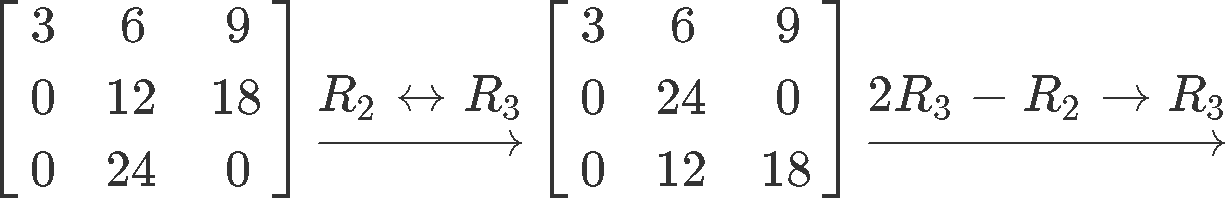Remember that you do not have to put the matrix in reduced echelon form, just to a general echelon form like the one above is fine, and so, we can now count the number of pivots: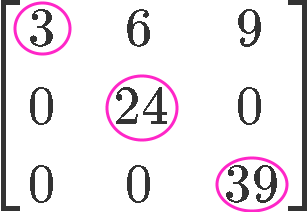Since there are 3 pivots in the echelon form matrix, then the rank of the matrix is 3.

#### Example 2

Find a basis for the subspace spanned by the given vectors. What is the dimension of the subspace?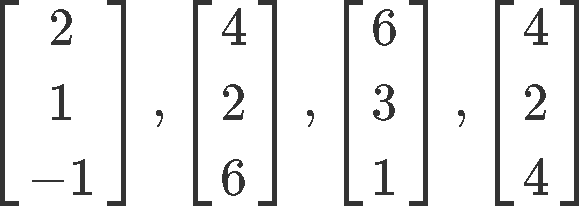First let us understand what is being asked in this problem: The basis for the subspace spanned by the given vectors is what we call the column space, since the column space is an array of vectors from a linear combination (in other words the column space of a span). Therefore, this problem is asking us to find the basis for the column space, and then, to find the dimension of the column space which is the rank.

In order to find the basis and rank of the column vectors provided we put them together into a matrix and row reduce them into an echelon form: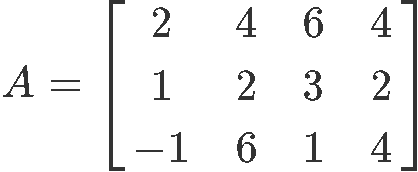Row reducing into echelon form: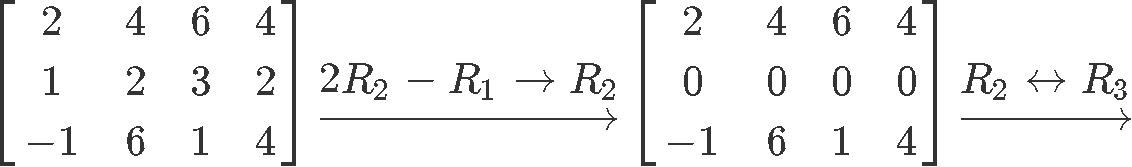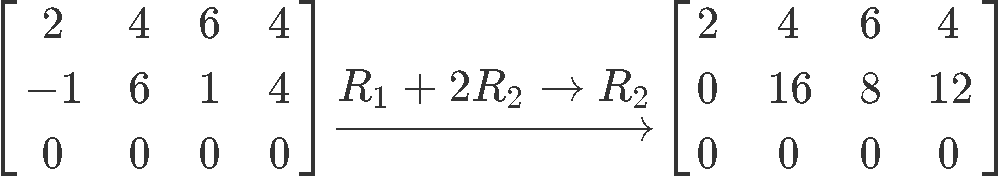And so, we circle the columns on the echelon form containing pivots and do the same in the original matrix: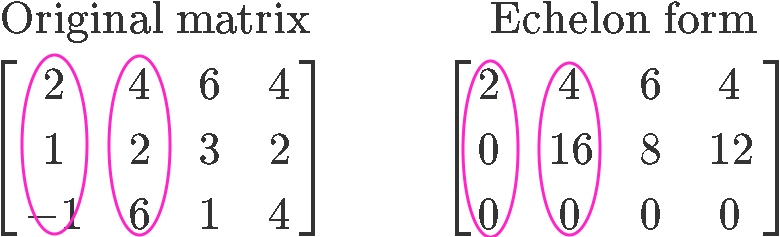These columns are the column vectors that form the basis for the column space of $A$: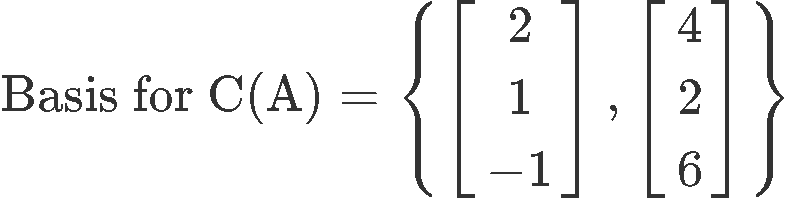And since there are two pivots in the echelon form matrix, then the dimension of the subspace of the provided column vectors, which is the dimension of the column space of the matrix or rank, is equal to 2.

#### Example 3

Find the dimension of the null space of $A$ if:Remember we call the dimension of null space nullity. In order to find the nullity of matrix $A$ we solve the matrix equation $Ax=0$ in order find how many free variables are contained in the vector $x$. First we set up the equation: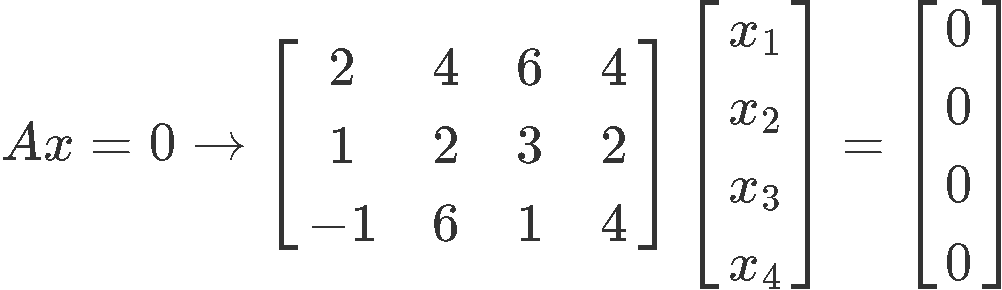Where the column vector $x$ contains 4 entries because $A$ has 4 columns, and the resultant zero vector must have 3 entries because $A$ has 3 rows (this is all following the rules for matrix multiplication). Using equation 12 we form the augmented matrix below: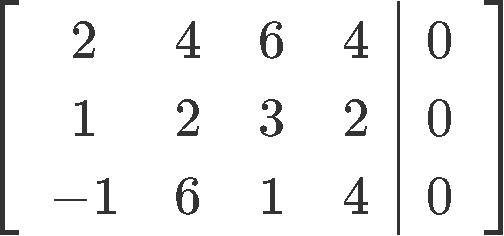And we row reduce to reduced echelon form: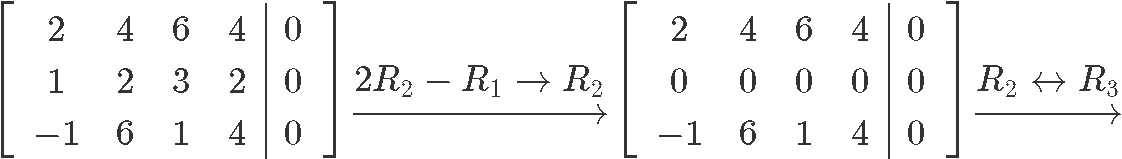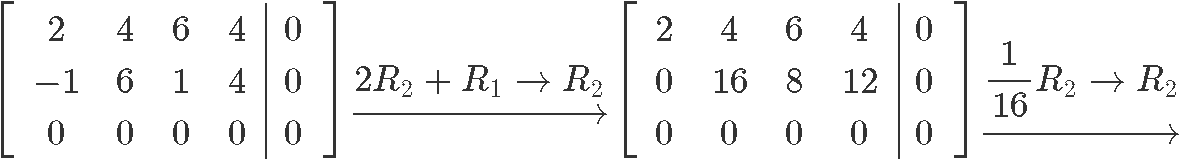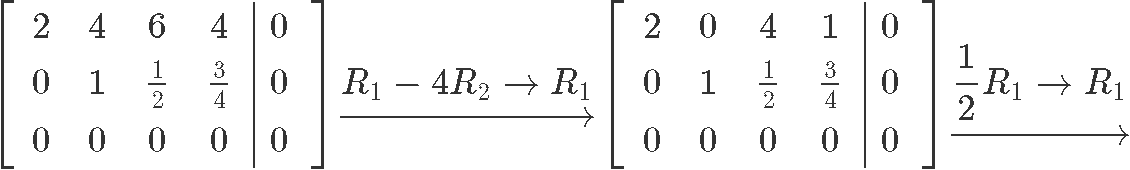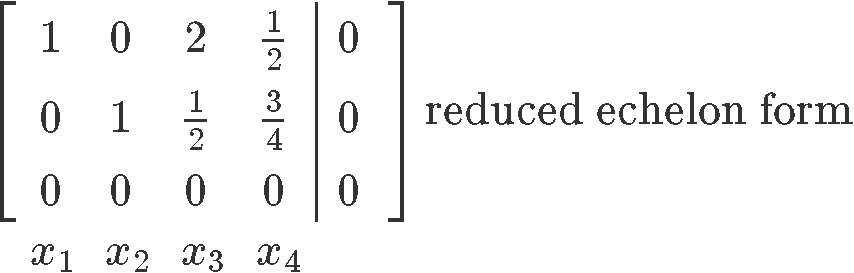Remember that the free variables are those which do not have a pivot on the reduced echelon form, therefore, $x_3$ and $x_4$ are free variables. Since there are two free variables, the dimension of the null space, or nullity is equal to 2.

#### Example 4

If you are given the matrix $A$ and its echelon form as shown below, find the basis for the column space, and find the rank and the dimensions of the null space.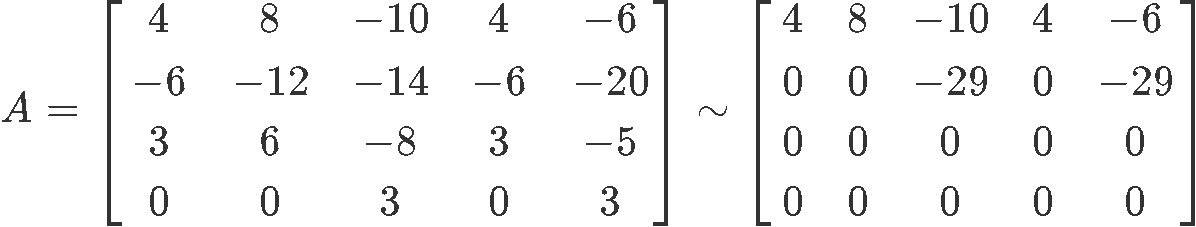Let us separate the solutions of this problem in three parts for the elements that are needed to be solved:

• Find the basis of the column space of A:
Since we already have the echelon form of the matrix, we just need to find the pivots, circle the columns with pivots on it and do the same in the original matrix $A$.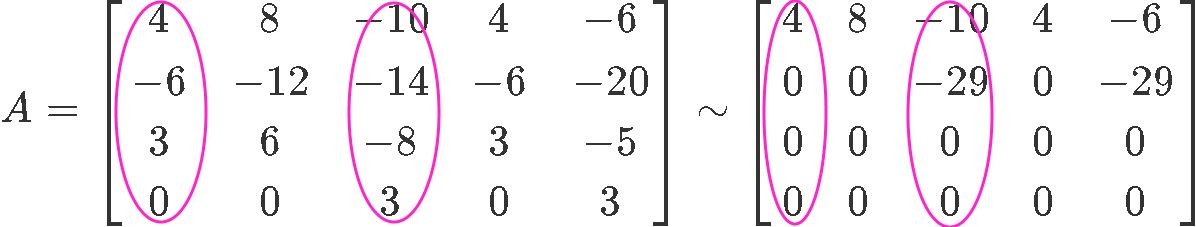These columns (from the original matrix $A$) are the column vectors which form the basis for the column space of $A$: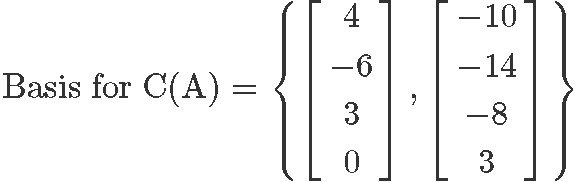• Find the rank:
To find the rank we just count the vectors in the basis of the column space. Since there are two vectors as shown in equation 17, the dimension of the column space of $A$ (rank of $A$) is equal to:

rank$(A) = 2$

• Find the dimensions of the null space (nullity):
Now this part of the problem can be done in two ways, either take matrix $A$ and solve the matrix equation $Ax=0$ to find the amount of free variables or simply, use the rank-nullity theorem from equation 2 to find the nullity of $A$ easily. Lets use this second method since is much faster!

rank$(A) \, + \,$nullity$(A) = n$

Where: rank $(A) = 2 \,$and$\, n=5, \,$therefore:

rank$(A) \, + \,$nullity$(A) = 2 \, + \,$nullity$(A) = 5$

nullity$(A) = 5 -2 = 3$

Notice that we knew the rank of $A$ from the second part of the problem, and then we knew $n$ because is the number of columns in the matrix, which for matrix $A$ is 5.

#### Example 5

Let $A$ be a $m \times n$ matrix, where the rank of $A$ is $p$. Then, what is the dimension of the null space of $A$?
We use the rank-nullity theorem once more and obtain nullity:

rank$(A) \, + \,$nullity$(A) = n$

Where: rank $(A) = p$

rank$(A) \, + \,$nullity$(A) = p \, + \,$nullity$(A) = n$

nullity$(A) = n - p$

Since nullity is the dimension of the null space of the matrix, this cannot be a negative number, therefore:

nullity$(A) = n - p \geq 0$

***

Having solved exercises problems all we are left to do is to recommend you a few interesting and useful resources from the internet. The next link on dimension, rank and determinants gives you a few extra examples and very clear concepts on our topic of today; also, this linear algebra review on these topics comes compact and complete and could be very useful in your independent studies.

This is the end of our lesson for today, see you in the next one!
Dimension of a Subspace
The dimension of a non-zero subspace $S$ (usually denoted as dim $S$), is the # of vectors in any basis for $S$. Since the null space and column space is a subspace, we can find their dimensions.

Note: Dimension of the column space = rank

Finding the Rank of a matrix:
1. Find the basis for the column space
2. Count the # of vectors in the basis. That is the rank.
Shortcut: Count the # of pivots in the matrix

Finding the dimensions of the null space:
1. Find the basis for the null space
2. Count the # of vectors in the basis. That is the dimension.
Shortcut: Count the # of free variables in the matrix.

The Rank Theorem
If a matrix $A$ has $n$ columns, then rank $A+$ dim $N(A) = n$.